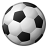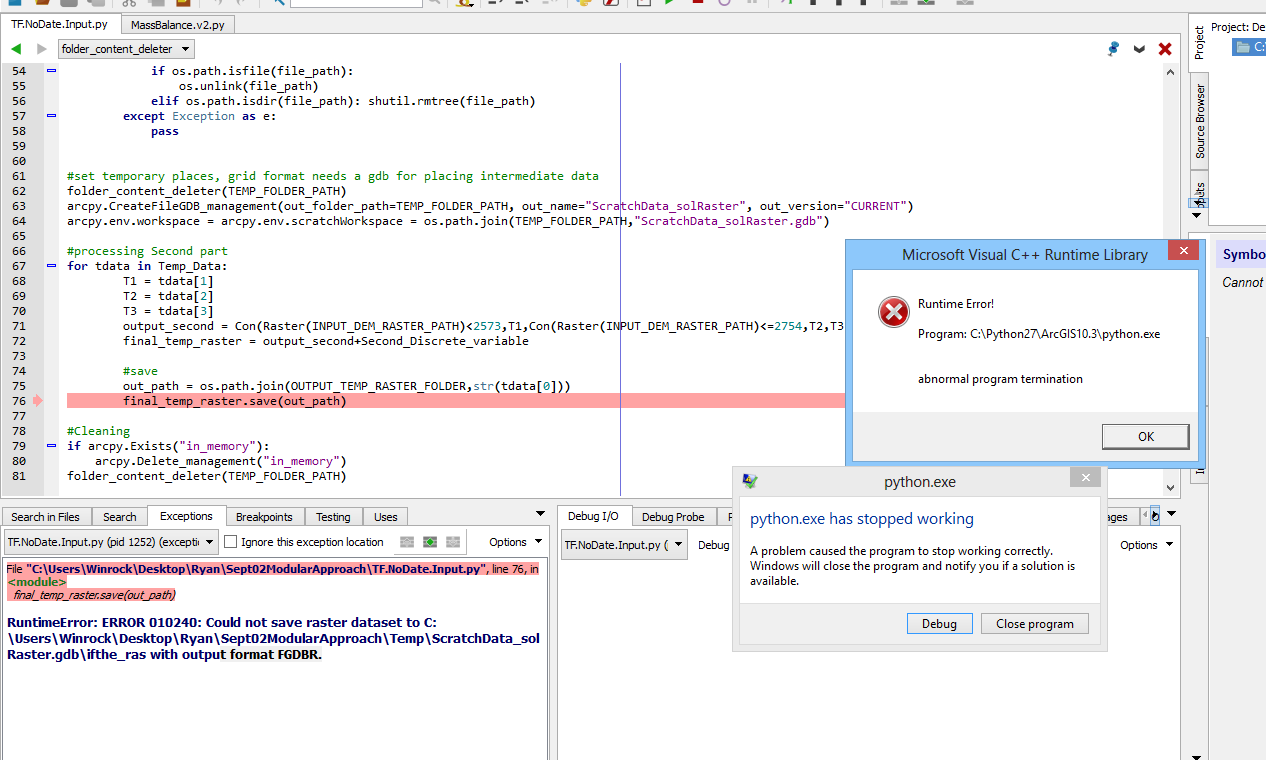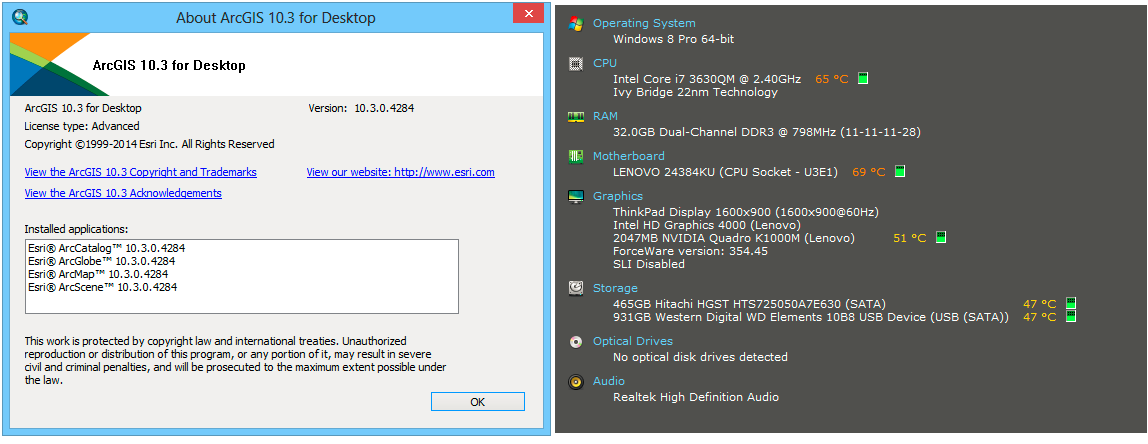# RuntimeError: ERROR 010240 in Con operation in arcpy

2345
8
09-09-2016 08:16 PMNew Contributor III

I am dabbling with the resolution of the error says `RuntimeError: ERROR 010240: Could not save raster dataset to` as attached as a screen shot. I am working with a DEM file and trying to generate raster based on the conditional values from an excel file. As the excel file has about 15000 row, I need to generate about 15000 raster. I am very much disappointed when the script takes long time and at last it fails every time at different point and this has been happening from the yesterday.

I am just reading date and associated 3 temperature values from the excel file and applying con operation on DEM arcgis grid raster to generate another raster. This process is repeated for all the dates i.e. rows in the excel file as attached.

My script is a below

``#!/usr/bin/python# -*- coding: utf-8 -*-import arcpyimport osimport sysimport shutilfrom arcpy.sa import Confrom arcpy.sa import Rasterfrom openpyxl import load_workbookarcpy.env.overwriteOutput = Truearcpy.CheckOutExtension('spatial')INPUT_TEMP_EXCEL_PATH = \    r"C:\Users\Winrock\Desktop\Ryan\Sept02ModularApproach\Temperature Model Data.xlsx"  # arcpy.GetParameterAsText(0)INPUT_DEM_RASTER_PATH = \    r"C:\Users\Winrock\Desktop\Ryan\Sept02ModularApproach\DEM\dem_clip_11"  # arcpy.GetParameterAsText(1)Second_Discrete_variable = 10  # arcpy.GetParameterAsText(2)OUTPUT_TEMP_RASTER_FOLDER = \    r"C:\Users\Winrock\Desktop\Ryan\Sept02ModularApproach\OutputRaster"  # arcpy.GetParameterAsText(3)TEMP_FOLDER_PATH = \    r"C:\Users\Winrock\Desktop\Ryan\Sept02ModularApproach\Temp"  # arcpy.GetParameterAsText(4)Second_Discrete_variable = float(Second_Discrete_variable)Temp_Data = []# Loading temperature datatemp_wb = load_workbook(filename=INPUT_TEMP_EXCEL_PATH, read_only=True)temp_ws = temp_wb[temp_wb.sheetnames]for row in temp_ws.rows:    rw = [cell.value for cell in row]    Temp_Data.append(rw)Temp_Data = Temp_Data[1:]# Folder content deleterdef folder_content_deleter(folder_path):    for the_file in os.listdir(folder_path):        file_path = os.path.join(folder_path, the_file)        try:            if os.path.isfile(file_path):                os.unlink(file_path)            elif os.path.isdir(file_path):                shutil.rmtree(file_path)        except Exception, e:            pass# set temporary places, grid format needs a gdb for placing intermediate datafolder_content_deleter(TEMP_FOLDER_PATH)arcpy.CreateFileGDB_management(out_folder_path=TEMP_FOLDER_PATH,                               out_name='ScratchData_solRaster',                               out_version='CURRENT')arcpy.env.workspace = arcpy.env.scratchWorkspace = \    os.path.join(TEMP_FOLDER_PATH, 'ScratchData_solRaster.gdb')# processing Second partfor tdata in Temp_Data:    T1 = tdata    T2 = tdata    T3 = tdata    output_second = Con(Raster(INPUT_DEM_RASTER_PATH) < 2573, T1,                        Con(Raster(INPUT_DEM_RASTER_PATH) <= 2754, T2,                        T3))    final_temp_raster = output_second + Second_Discrete_variable        # save    out_path = os.path.join(OUTPUT_TEMP_RASTER_FOLDER, str(tdata))    final_temp_raster.save(out_path)# Cleaningif arcpy.Exists('in_memory'):    arcpy.Delete_management('in_memory')folder_content_deleter(TEMP_FOLDER_PATH)‍‍‍‍‍‍‍‍‍‍‍‍‍‍‍‍‍‍‍‍‍‍‍‍‍‍‍‍‍‍‍‍‍‍‍‍‍‍‍‍‍‍‍‍‍‍‍‍‍‍‍‍‍‍‍‍‍‍‍‍‍‍‍‍‍‍‍‍‍‍‍‍‍‍‍‍‍‍‍‍‍‍‍``

My error in gist is-

RuntimeError: ERROR 010240: Could not save raster dataset to C:\Users\Winrock\Desktop\Ryan\Sept02ModularApproach\Temp\ScratchData_solRaster.gdb\ifthe_ras with output format FGDBR

The excel file I am using is  https://www.dropbox.com/s/qacfhipo4ry7o2b/Temperature%20Model%20Data.xlsx?dl=0

My error-N.B. I tried several thread some of them are-

System specification:---------------------------------------------------------------------------------------------------------------------------------------------------------------

Update:

*I tried with different scratch and current workspace

*I tried with arcpy.gp.Times_sa(it stops after processing almost 3000 rasters) and arcpy.sa.Times(it stops after processing almost 1070 rasters)

*I tried with arcpy.TestSchemaLock

*I tried with setting output to tif format too.

I see that it stops and raises error when it processed and output exact number of 1070 grid files.

Tags (4)
1 Solution

Accepted SolutionsNew Contributor III

Thanks all Dan and Xander,

Al last I solved the problem!

My understanding that solved the problem:

1. Use separate scratch and output work-space
2. Using multiprocessing is imperative if raster to process is so many(I am obliged to Dan for this concept)- it is really helpful though slow in comparison to the other multiprocessing tasks- esri engineers can tell more about it.
4. Always clean the garbage in each work-space.
5. If given multiple raster to process then arcpy.gp.Times_sa can handle more than the arcpy.sa.Times can handle.Really it is absurd that deprecated tool is more powerful that the new one (i call it a nascent babe or at least an apple of Sodom)

My understanding about arcpy in raster processing at least in their grid format:

• Arcpy can not handle task that involves more than 3000 raster at a stretch to process.

Below is my script so far:

``#!/usr/bin/python# -*- coding: utf-8 -*-#Author:Shariful Islam#Contact: msi_g@yahoo.comimport arcpy,os,shutil,multiprocessing,refrom arcpy.sa import Confrom arcpy.sa import Rasterarcpy.env.overwriteOutput = Truearcpy.CheckOutExtension("spatial")try:    from openpyxl import load_workbookexcept:    raise Exception("install the openpyxl module in your python system")#================================================================================================================##change below as it suits your system. try to make short all path below e.g. C:\OutputRsater  D:\InputTempFile.xlsx#just change the paths i.e. right side of the equations below nothing else- be careful.INPUT_TEMP_EXCEL_PATH =  r"C:\test\Temprt1.xlsx"INPUT_DEM_RASTER_PATH =  r"C:\test\dem_clip_11"OUTPUT_TEMP_RASTER_FOLDER = r"C:\test\myout1"TEMP_FOLDER_PATH = r"C:\test\mytemp"#do not change below from here#================================================================================================================#Temp_Data = []#Loading temperature datatemp_wb = load_workbook(filename=INPUT_TEMP_EXCEL_PATH, read_only=True)temp_ws = temp_wb[temp_wb.sheetnames]for row in temp_ws.rows:    d = []    if len(row)>3:        for cell in row:            if cell.value == None:                pass            elif cell.value == 0:                d.append(0.000000)            elif isinstance(cell.value, float):                d.append(round(cell.value,6))            else:                d.append(cell.value)        Temp_Data.append(d)#process collected excel dataTemp_Data= Temp_Data[1:]seen = set()Temp_Data = [x for x in Temp_Data if x not in seen and not seen.add(x)]# removing duplicate date        #Folder content deleterdef folder_content_deleter(folder_path):    for the_file in os.listdir(folder_path):        file_path = os.path.join(folder_path, the_file)        try:            if os.path.isfile(file_path):                os.unlink(file_path)            elif os.path.isdir(file_path): shutil.rmtree(file_path)        except Exception as e:            pass#folder deleterdef purge(dirpth, pattern):    for f in os.listdir(dirpth):        if re.search(pattern, f):            pth = os.path.join(dirpth, f)            shutil.rmtree(pth, ignore_errors=True) #gdb content deleterdef gdb_content_deleter(wrkspc):    for r,d,fls in arcpy.da.Walk(wrkspc, datatype="FeatureClass"):        for f in fls:            print f            try:                arcpy.Delete_management(os.path.join(r,f))            except:                pass #copy and group by yeardef grouperByYear(input_folder_path, output_folder_path):    for dirpath, dirnames, filenames in arcpy.da.Walk(input_folder_path, topdown=True, datatype="RasterDataset", type="GRID"):        for filename in filenames:            out_folder_name = re.findall(r'(?<=\g)\d{4}', filename)            out_folder_path = os.path.join(output_folder_path,out_folder_name)            if not os.path.exists(out_folder_path):                print "Creating and populating folder for year %s ......"%out_folder_name                os.mkdir(out_folder_path)            in_data = os.path.join(dirpath,filename)            ou_feature_name = 'g'+re.findall(r'(?<=\g\d{4})\d{4}\$',filename)            out_data = os.path.join(out_folder_path,ou_feature_name)            arcpy.Copy_management(in_data, out_data)#processing Second partdef times_worker(times_range_list):    #set temporary places, grid format needs a gdb for placing intermediate data    #folder_content_deleter(TEMP_FOLDER_PATH)    scratch_db_name = "Scratch_"+str(times_range_list)    arcpy.CreateFileGDB_management(out_folder_path=TEMP_FOLDER_PATH, out_name=scratch_db_name, out_version="CURRENT")    scr_db = os.path.join(TEMP_FOLDER_PATH,scratch_db_name+".gdb")    arcpy.env.scratchWorkspace = scr_db        #set output db    out_db_name = "RData_"+str(times_range_list)    #arcpy.CreateFileGDB_management(out_folder_path=OUTPUT_TEMP_RASTER_FOLDER, out_name=out_db_name, out_version="CURRENT")    #out_db = os.path.join(OUTPUT_TEMP_RASTER_FOLDER,out_db_name+".gdb")    out_db = os.path.join(OUTPUT_TEMP_RASTER_FOLDER,out_db_name)    if not os.path.exists(out_db):os.mkdir(out_db)    arcpy.env.workspace  = out_db        for tdata in times_range_list:            T1 = float('%.6f'%tdata)            T2 = float('%.6f'%tdata)            T3 = float('%.6f'%tdata)            out_path = os.path.join(out_db,'g'+str(tdata))            outRast_name = "in_memory\\%s"%out_db_name            arcpy.MakeRasterLayer_management(INPUT_DEM_RASTER_PATH,outRast_name)            output_second = Con(Raster(outRast_name)<2573,T1,Con(Raster(outRast_name)<=2754,T2,T3))            final_temp_raster = output_second                        #save            final_temp_raster.save(out_path)                        #cleaning            gdb_content_deleter(scr_db)            def main(cu, worker, d_range):    pool = multiprocessing.Pool(cu)    pool.map(worker,d_range,1)    pool.close()    pool.join()                if __name__ == '__main__':    core_usage = 5     chunk_size = 1000    needed_cpu = int(round((len(Temp_Data)/chunk_size),0)+1)    offsetter = list(divmod(needed_cpu, core_usage))    cpu_distribution = [core_usage]*offsetter+[offsetter]    cpu_distribution = [cp for cp in cpu_distribution if cp!=0]#just remove zero    temp_data_range = [Temp_Data[i:i+chunk_size] for i in range(0,len(Temp_Data),chunk_size)]    print r"Doing raster math. It may take upto 3-7 hours even and may use your cpu at the heighest.\          So stop using your cpu fo this time. Go and enjoy elsewhere, let me do the job for you!.........."        loopcnt = 0    for cpu in cpu_distribution:        temp_data_range_splitted = temp_data_range[loopcnt:loopcnt+cpu]        if len(temp_data_range_splitted)>0:            main(cpu, times_worker, temp_data_range_splitted)            loopcnt+=cpu                #Cleaning        if arcpy.Exists("in_memory"):            arcpy.Delete_management("in_memory")        folder_content_deleter(TEMP_FOLDER_PATH)        #group by year    print "\nGrouping raster math output by year for you. It may take 1-2 hours at best.So stay tuned!........\n"    grouperByYear(OUTPUT_TEMP_RASTER_FOLDER, OUTPUT_TEMP_RASTER_FOLDER)        #delete unnecessary folders    print "\nCleaning all unnecessary files........\n"    purge(OUTPUT_TEMP_RASTER_FOLDER, r'RData_[0-9]{8}')        print r"All job finished! Now you are ready for the processing:)"‍‍‍‍‍‍‍‍‍‍‍‍‍‍‍‍‍‍‍‍‍‍‍‍‍‍‍‍‍‍‍‍‍‍‍‍‍‍‍‍‍‍‍‍‍‍‍‍‍‍‍‍‍‍‍‍‍‍‍‍‍‍‍‍‍‍‍‍‍‍‍‍‍‍‍‍‍‍‍‍‍‍‍‍‍‍‍‍‍‍‍‍‍‍‍‍‍‍‍‍‍‍‍‍‍‍‍‍‍‍‍‍‍‍‍‍‍‍‍‍‍‍‍‍‍‍‍‍‍‍‍‍‍‍‍‍‍‍‍‍‍‍‍‍‍‍‍‍‍‍‍‍‍‍‍‍‍‍‍‍‍‍‍‍‍‍‍‍‍‍‍‍‍‍‍‍‍‍‍‍‍‍‍‍‍‍‍‍‍‍‍‍‍‍‍‍‍‍‍‍‍‍‍‍‍‍‍‍‍‍‍‍‍‍‍‍‍‍‍‍‍‍‍‍‍‍‍‍‍‍‍‍‍‍‍‍‍‍‍‍‍‍‍‍‍‍‍‍‍‍‍‍‍‍‍‍‍‍‍‍‍‍‍‍‍‍‍‍‍‍‍‍‍‍‍‍‍‍‍‍‍‍‍‍‍‍‍‍‍‍‍‍‍‍‍‍‍‍‍‍‍‍‍‍‍‍‍‍‍‍‍‍‍‍‍‍‍‍‍‍‍‍‍‍‍‍‍‍‍‍‍‍‍‍‍‍‍‍‍‍‍‍‍‍‍‍‍‍‍‍‍‍‍‍‍‍‍‍‍‍‍‍‍‍‍‍‍‍‍‍‍‍‍‍‍‍‍‍‍‍‍‍‍‍‍‍‍‍‍‍‍‍‍‍‍‍‍‍‍‍‍‍‍‍‍‍‍‍‍‍‍‍‍‍‍‍‍‍‍‍‍‍‍‍‍‍‍‍‍‍‍‍‍‍‍‍‍‍‍‍‍‍‍‍‍‍‍‍‍‍‍‍‍‍‍‍‍‍‍‍‍‍‍‍‍‍‍‍‍‍‍‍‍‍‍‍‍‍‍‍‍‍‍‍‍‍‍‍‍‍‍‍‍‍‍‍‍‍‍‍‍‍‍‍‍‍‍‍‍‍‍‍‍‍‍‍‍‍‍‍‍‍‍‍‍‍‍‍‍‍‍‍‍‍‍‍‍‍‍‍‍‍‍‍‍‍‍‍‍‍‍‍‍‍‍‍‍‍‍‍‍‍‍‍‍‍‍‍‍‍‍‍‍‍‍‍‍‍‍‍‍‍‍‍‍‍‍‍‍‍‍‍‍‍‍‍‍‍‍‍‍‍‍‍‍‍‍‍‍‍‍‍‍‍‍‍‍‍‍‍‍‍‍‍‍‍‍‍‍‍‍‍‍‍‍‍‍‍‍‍‍‍‍‍‍‍‍‍‍‍‍‍‍‍‍‍‍‍‍‍‍‍‍‍‍‍‍‍‍‍‍‍‍‍‍‍‍‍‍‍‍‍‍‍‍‍‍‍‍‍‍‍‍‍‍‍‍‍‍‍‍‍‍‍‍‍‍‍‍‍‍‍‍‍‍‍‍‍‍‍‍‍‍‍‍‍‍‍‍‍‍‍‍‍‍‍‍‍‍‍‍‍‍‍‍‍‍‍‍‍‍‍‍‍‍‍‍‍‍‍‍‍‍‍‍‍‍‍‍‍‍‍‍‍‍‍‍‍‍‍‍‍‍‍‍‍‍‍‍‍‍‍‍‍‍‍‍‍‍‍‍‍‍‍‍‍‍‍‍‍‍‍‍‍‍‍‍‍‍‍‍‍‍‍‍‍‍‍‍‍‍‍‍‍‍‍‍‍‍‍‍‍‍‍‍‍‍‍‍‍‍‍‍‍‍‍‍‍‍‍‍‍‍‍‍‍‍‍‍‍‍‍‍‍‍‍‍‍‍‍‍‍‍‍‍‍‍‍‍‍‍‍‍‍‍‍‍‍‍‍‍‍‍‍‍‍‍‍‍‍‍‍‍‍‍‍‍‍‍‍‍‍‍‍‍‍‍‍‍‍‍‍‍‍‍‍‍‍‍‍‍‍‍‍‍‍‍‍‍‍‍‍‍‍‍‍‍‍‍‍‍‍‍‍‍‍‍‍‍‍‍‍‍‍‍‍‍‍‍‍‍‍‍‍‍‍‍‍‍‍‍‍‍‍‍‍‍‍‍‍‍‍‍‍‍‍‍‍‍‍‍‍‍‍‍‍‍‍‍‍‍‍‍‍‍‍‍‍‍‍‍‍‍‍‍‍‍‍‍‍‍‍‍‍‍‍‍‍‍‍‍‍‍‍‍‍‍‍‍‍‍‍‍‍‍‍‍‍‍‍‍‍‍‍‍‍‍‍‍‍‍‍‍‍‍‍‍‍‍‍‍‍‍‍‍‍‍‍‍‍‍‍‍‍‍‍‍‍‍‍‍‍‍‍‍‍‍‍‍‍‍‍‍‍‍‍‍‍‍‍‍‍‍‍‍‍‍‍‍‍‍‍‍‍‍‍‍‍‍‍‍‍‍‍‍‍‍‍‍‍‍‍‍‍‍‍‍‍‍‍‍‍‍‍‍‍‍‍‍‍‍‍‍‍‍‍‍‍‍‍‍‍‍‍‍‍‍‍‍‍‍‍‍‍‍‍‍‍‍‍‍‍‍‍‍‍‍‍‍‍‍‍‍‍‍‍‍‍‍‍‍‍‍‍‍‍‍‍‍‍‍‍‍‍‍‍‍‍‍‍‍‍‍‍‍‍‍‍‍‍‍‍‍‍‍‍‍‍‍‍‍‍‍‍‍‍‍‍‍‍‍‍‍‍‍‍‍‍‍‍‍‍‍‍‍‍‍‍‍‍‍‍‍‍‍‍‍‍‍‍‍‍‍‍‍‍‍‍‍‍‍‍‍‍‍‍‍‍‍‍‍‍‍‍‍‍‍‍‍‍‍‍‍‍‍‍‍‍‍‍‍‍‍‍‍‍‍‍‍‍‍‍‍‍‍‍‍‍‍‍‍‍‍‍‍‍‍‍‍‍‍‍‍‍‍‍‍‍‍‍‍‍‍‍‍‍‍‍‍‍‍‍‍‍‍‍``
8 RepliesbyMVP Esteemed Contributor

you are being overly optimistic in processing that many rasters within the 4 GB limits without deleting stuff as you go.  As a suggestion, I would get the spreadsheet into an alternate readable format and dispense with its overhead. As well you path is long and potentially causing problems... try something simple like c:\test and a shorter grid name... it appears you are using grids which have specific filename requirements, so try 'X' or if you want to use another format 'X.tif'.  Keep things simple .... process in smaller batches ... switch to 64 bit background processing if possible or more to python 3.4.x and arcgis pro if you want to use all your available memory rather than tiling or processing in batches.

Try some of the above, monitor your system resources as you go, and you will narrow down your issue.  You could profile your script to find out where memory is being consumed (there are modules in the Anaconda distribution for ArcGIS Pro 1.3 that will help with this.)...   Good luck.byEsri Esteemed Contributor

I would definitely store the Excel data in a table (fgdb) and also trying to store 15000 grids in a single folder will certainly yield errors. Also avoid long folder structures and name the output raster to be sure that the output name does not exceed limits.byMVP Esteemed Contributor

The number of rasters isn't the issue ( https://community.esri.com/thread/173806 ).

It is file management, you aren't cleaning up as you go and as Xander points out... storing 15000 grids... do you really need them all and do you need them all at once???

The one thing you failed to mention is whether you ran it in steps... bites, portions... then append the pieces or work with their resultant.New Contributor III

Thanks Dan!

At last I tried to implement some of your advice. Is there any problem to read excel into a list and using this list later in the geoprocessing? I transferred all in root folder and tried to delete all the intermediate files after it is being used.  I tried to save rasters as tif file and checked if align with the naming convention. In fact each raster name length is 8 e.g. 19120205  .But all with no success.

``import arcpy,os,sys,shutil,time,csvfrom arcpy.sa import Confrom arcpy.sa import Rasterfrom openpyxl import load_workbookarcpy.env.overwriteOutput = Truearcpy.CheckOutExtension("spatial")INPUT_TEMP_EXCEL_PATH = (#arcpy.GetParameterAsText(0)                        r"C:\test\Temprt.xlsx"                         )INPUT_DEM_RASTER_PATH = (#arcpy.GetParameterAsText(1)                             r"C:\test\dem_clip_11"                         )Second_Discrete_variable = (#arcpy.GetParameterAsText(2)                               1                            )OUTPUT_TEMP_RASTER_FOLDER = (#arcpy.GetParameterAsText(3)                          r"C:\test\myout"                         )TEMP_FOLDER_PATH = (#arcpy.GetParameterAsText(4)                         r"C:\test\mytemp"                         )Second_Discrete_variable = float('%.6f'%Second_Discrete_variable)Temp_Data = []#Loading temperature datatemp_wb = load_workbook(filename=INPUT_TEMP_EXCEL_PATH, read_only=True)temp_ws = temp_wb[temp_wb.sheetnames]for row in temp_ws.rows:    d = []    for cell in row:        if cell.value == None:            pass        elif cell.value == 0:            d.append(0.000000)        elif isinstance(cell.value, float):            d.append(round(cell.value,6))        else:            d.append(cell.value)    Temp_Data.append(d)#process collected excel dataTemp_Data= Temp_Data[1:]seen = set()Temp_Data = [x for x in Temp_Data if x not in seen and not seen.add(x)]# removing duplicate date        #Folder content deleterdef folder_content_deleter(folder_path):    for the_file in os.listdir(folder_path):        file_path = os.path.join(folder_path, the_file)        try:            if os.path.isfile(file_path):                os.unlink(file_path)            elif os.path.isdir(file_path): shutil.rmtree(file_path)        except Exception as e:            pass #gdb content deleterdef gdb_content_deleter(wrkspc):    for r,d,fls in arcpy.da.Walk(wrkspc, datatype="FeatureClass"):        for f in fls:            print f            try:                arcpy.Delete_management(os.path.join(r,f))                print "deleted scratch"            except:                pass            #set temporary places, grid format needs a gdb for placing intermediate datafolder_content_deleter(TEMP_FOLDER_PATH)arcpy.CreateFileGDB_management(out_folder_path=TEMP_FOLDER_PATH, out_name="SDB_SR", out_version="CURRENT")scr_db = os.path.join(TEMP_FOLDER_PATH,"SDB_SR.gdb")arcpy.env.scratchWorkspace = scr_db#processing Second partcounter = 0for tdata in Temp_Data:        T1 = float('%.6f'%tdata)        T2 = float('%.6f'%tdata)        T3 = float('%.6f'%tdata)        out_folder_name = str(tdata)[0:4]        out_folder = os.path.join(OUTPUT_TEMP_RASTER_FOLDER,out_folder_name)        if not os.path.exists(out_folder):os.mkdir(out_folder)        arcpy.env.workspace  = out_folder        out_path = os.path.join(out_folder,str(tdata)+'.tif')        output_second = Con(Raster(INPUT_DEM_RASTER_PATH)<2573,T1,Con(Raster(INPUT_DEM_RASTER_PATH)<=2754,T2,T3))                #apply map algebra if feature exists        try:            final_temp_raster = arcpy.gp.Times_sa(output_second, str(Second_Discrete_variable), "in_memory\\test_"+str(counter))            qq = final_temp_raster.getoutput(0)            qq=Raster(qq)            final_temp_raster = qq            except:            pass            try:                    #check if wait is of use                time.sleep(15)                final_temp_raster = arcpy.gp.Times_sa(output_second, str(Second_Discrete_variable), "in_memory\\test_"+str(counter))                qq = final_temp_raster.getoutput(0)                qq=Raster(qq)                final_temp_raster = qq            except:                pass                with open(os.path.join(TEMP_FOLDER_PATH,'error.csv'), 'ab') as error_file:                    writr = csv.writer(error_file)                    writr.writerow(tdata)        counter+=1         #save        final_temp_raster.save(out_path)                #cleaning        if arcpy.Exists("in_memory"):            arcpy.Delete_management("in_memory")        gdb_content_deleter(scr_db)#Cleaningif arcpy.Exists("in_memory"):    arcpy.Delete_management("in_memory")folder_content_deleter(TEMP_FOLDER_PATH)‍‍‍‍‍‍‍‍‍‍‍‍‍‍‍‍‍‍‍‍‍‍‍‍‍‍‍‍‍‍‍‍‍‍‍‍‍‍‍‍‍‍‍‍‍‍‍‍‍‍‍‍‍‍‍‍‍‍‍‍‍‍‍‍‍‍‍‍‍‍‍‍‍‍‍‍‍‍‍‍‍‍‍‍‍‍‍‍‍‍‍‍‍‍‍‍‍‍‍‍‍‍‍‍‍‍‍‍‍‍‍‍‍‍‍‍‍‍‍‍‍‍‍‍‍‍``byMVP Esteemed Contributor

and I can't tell from the code, but grid names can't begin with a number

A copy of the full error message again

There is no indication you have run it on fewer inputs..byMVP Esteemed Contributor

also... can you add some print statements within the process to monitor what is being used as variable values and  filenamesNew Contributor III

Thanks all Dan and Xander,

Al last I solved the problem!

My understanding that solved the problem:

1. Use separate scratch and output work-space
2. Using multiprocessing is imperative if raster to process is so many(I am obliged to Dan for this concept)- it is really helpful though slow in comparison to the other multiprocessing tasks- esri engineers can tell more about it.
4. Always clean the garbage in each work-space.
5. If given multiple raster to process then arcpy.gp.Times_sa can handle more than the arcpy.sa.Times can handle.Really it is absurd that deprecated tool is more powerful that the new one (i call it a nascent babe or at least an apple of Sodom)

My understanding about arcpy in raster processing at least in their grid format:

• Arcpy can not handle task that involves more than 3000 raster at a stretch to process.

Below is my script so far:

``#!/usr/bin/python# -*- coding: utf-8 -*-#Author:Shariful Islam#Contact: msi_g@yahoo.comimport arcpy,os,shutil,multiprocessing,refrom arcpy.sa import Confrom arcpy.sa import Rasterarcpy.env.overwriteOutput = Truearcpy.CheckOutExtension("spatial")try:    from openpyxl import load_workbookexcept:    raise Exception("install the openpyxl module in your python system")#================================================================================================================##change below as it suits your system. try to make short all path below e.g. C:\OutputRsater  D:\InputTempFile.xlsx#just change the paths i.e. right side of the equations below nothing else- be careful.INPUT_TEMP_EXCEL_PATH =  r"C:\test\Temprt1.xlsx"INPUT_DEM_RASTER_PATH =  r"C:\test\dem_clip_11"OUTPUT_TEMP_RASTER_FOLDER = r"C:\test\myout1"TEMP_FOLDER_PATH = r"C:\test\mytemp"#do not change below from here#================================================================================================================#Temp_Data = []#Loading temperature datatemp_wb = load_workbook(filename=INPUT_TEMP_EXCEL_PATH, read_only=True)temp_ws = temp_wb[temp_wb.sheetnames]for row in temp_ws.rows:    d = []    if len(row)>3:        for cell in row:            if cell.value == None:                pass            elif cell.value == 0:                d.append(0.000000)            elif isinstance(cell.value, float):                d.append(round(cell.value,6))            else:                d.append(cell.value)        Temp_Data.append(d)#process collected excel dataTemp_Data= Temp_Data[1:]seen = set()Temp_Data = [x for x in Temp_Data if x not in seen and not seen.add(x)]# removing duplicate date        #Folder content deleterdef folder_content_deleter(folder_path):    for the_file in os.listdir(folder_path):        file_path = os.path.join(folder_path, the_file)        try:            if os.path.isfile(file_path):                os.unlink(file_path)            elif os.path.isdir(file_path): shutil.rmtree(file_path)        except Exception as e:            pass#folder deleterdef purge(dirpth, pattern):    for f in os.listdir(dirpth):        if re.search(pattern, f):            pth = os.path.join(dirpth, f)            shutil.rmtree(pth, ignore_errors=True) #gdb content deleterdef gdb_content_deleter(wrkspc):    for r,d,fls in arcpy.da.Walk(wrkspc, datatype="FeatureClass"):        for f in fls:            print f            try:                arcpy.Delete_management(os.path.join(r,f))            except:                pass #copy and group by yeardef grouperByYear(input_folder_path, output_folder_path):    for dirpath, dirnames, filenames in arcpy.da.Walk(input_folder_path, topdown=True, datatype="RasterDataset", type="GRID"):        for filename in filenames:            out_folder_name = re.findall(r'(?<=\g)\d{4}', filename)            out_folder_path = os.path.join(output_folder_path,out_folder_name)            if not os.path.exists(out_folder_path):                print "Creating and populating folder for year %s ......"%out_folder_name                os.mkdir(out_folder_path)            in_data = os.path.join(dirpath,filename)            ou_feature_name = 'g'+re.findall(r'(?<=\g\d{4})\d{4}\$',filename)            out_data = os.path.join(out_folder_path,ou_feature_name)            arcpy.Copy_management(in_data, out_data)#processing Second partdef times_worker(times_range_list):    #set temporary places, grid format needs a gdb for placing intermediate data    #folder_content_deleter(TEMP_FOLDER_PATH)    scratch_db_name = "Scratch_"+str(times_range_list)    arcpy.CreateFileGDB_management(out_folder_path=TEMP_FOLDER_PATH, out_name=scratch_db_name, out_version="CURRENT")    scr_db = os.path.join(TEMP_FOLDER_PATH,scratch_db_name+".gdb")    arcpy.env.scratchWorkspace = scr_db        #set output db    out_db_name = "RData_"+str(times_range_list)    #arcpy.CreateFileGDB_management(out_folder_path=OUTPUT_TEMP_RASTER_FOLDER, out_name=out_db_name, out_version="CURRENT")    #out_db = os.path.join(OUTPUT_TEMP_RASTER_FOLDER,out_db_name+".gdb")    out_db = os.path.join(OUTPUT_TEMP_RASTER_FOLDER,out_db_name)    if not os.path.exists(out_db):os.mkdir(out_db)    arcpy.env.workspace  = out_db        for tdata in times_range_list:            T1 = float('%.6f'%tdata)            T2 = float('%.6f'%tdata)            T3 = float('%.6f'%tdata)            out_path = os.path.join(out_db,'g'+str(tdata))            outRast_name = "in_memory\\%s"%out_db_name            arcpy.MakeRasterLayer_management(INPUT_DEM_RASTER_PATH,outRast_name)            output_second = Con(Raster(outRast_name)<2573,T1,Con(Raster(outRast_name)<=2754,T2,T3))            final_temp_raster = output_second                        #save            final_temp_raster.save(out_path)                        #cleaning            gdb_content_deleter(scr_db)            def main(cu, worker, d_range):    pool = multiprocessing.Pool(cu)    pool.map(worker,d_range,1)    pool.close()    pool.join()                if __name__ == '__main__':    core_usage = 5     chunk_size = 1000    needed_cpu = int(round((len(Temp_Data)/chunk_size),0)+1)    offsetter = list(divmod(needed_cpu, core_usage))    cpu_distribution = [core_usage]*offsetter+[offsetter]    cpu_distribution = [cp for cp in cpu_distribution if cp!=0]#just remove zero    temp_data_range = [Temp_Data[i:i+chunk_size] for i in range(0,len(Temp_Data),chunk_size)]    print r"Doing raster math. It may take upto 3-7 hours even and may use your cpu at the heighest.\          So stop using your cpu fo this time. Go and enjoy elsewhere, let me do the job for you!.........."        loopcnt = 0    for cpu in cpu_distribution:        temp_data_range_splitted = temp_data_range[loopcnt:loopcnt+cpu]        if len(temp_data_range_splitted)>0:            main(cpu, times_worker, temp_data_range_splitted)            loopcnt+=cpu                #Cleaning        if arcpy.Exists("in_memory"):            arcpy.Delete_management("in_memory")        folder_content_deleter(TEMP_FOLDER_PATH)        #group by year    print "\nGrouping raster math output by year for you. It may take 1-2 hours at best.So stay tuned!........\n"    grouperByYear(OUTPUT_TEMP_RASTER_FOLDER, OUTPUT_TEMP_RASTER_FOLDER)        #delete unnecessary folders    print "\nCleaning all unnecessary files........\n"    purge(OUTPUT_TEMP_RASTER_FOLDER, r'RData_[0-9]{8}')        print r"All job finished! Now you are ready for the processing:)"‍‍‍‍‍‍‍‍‍‍‍‍‍‍‍‍‍‍‍‍‍‍‍‍‍‍‍‍‍‍‍‍‍‍‍‍‍‍‍‍‍‍‍‍‍‍‍‍‍‍‍‍‍‍‍‍‍‍‍‍‍‍‍‍‍‍‍‍‍‍‍‍‍‍‍‍‍‍‍‍‍‍‍‍‍‍‍‍‍‍‍‍‍‍‍‍‍‍‍‍‍‍‍‍‍‍‍‍‍‍‍‍‍‍‍‍‍‍‍‍‍‍‍‍‍‍‍‍‍‍‍‍‍‍‍‍‍‍‍‍‍‍‍‍‍‍‍‍‍‍‍‍‍‍‍‍‍‍‍‍‍‍‍‍‍‍‍‍‍‍‍‍‍‍‍‍‍‍‍‍‍‍‍‍‍‍‍‍‍‍‍‍‍‍‍‍‍‍‍‍‍‍‍‍‍‍‍‍‍‍‍‍‍‍‍‍‍‍‍‍‍‍‍‍‍‍‍‍‍‍‍‍‍‍‍‍‍‍‍‍‍‍‍‍‍‍‍‍‍‍‍‍‍‍‍‍‍‍‍‍‍‍‍‍‍‍‍‍‍‍‍‍‍‍‍‍‍‍‍‍‍‍‍‍‍‍‍‍‍‍‍‍‍‍‍‍‍‍‍‍‍‍‍‍‍‍‍‍‍‍‍‍‍‍‍‍‍‍‍‍‍‍‍‍‍‍‍‍‍‍‍‍‍‍‍‍‍‍‍‍‍‍‍‍‍‍‍‍‍‍‍‍‍‍‍‍‍‍‍‍‍‍‍‍‍‍‍‍‍‍‍‍‍‍‍‍‍‍‍‍‍‍‍‍‍‍‍‍‍‍‍‍‍‍‍‍‍‍‍‍‍‍‍‍‍‍‍‍‍‍‍‍‍‍‍‍‍‍‍‍‍‍‍‍‍‍‍‍‍‍‍‍‍‍‍‍‍‍‍‍‍‍‍‍‍‍‍‍‍‍‍‍‍‍‍‍‍‍‍‍‍‍‍‍‍‍‍‍‍‍‍‍‍‍‍‍‍‍‍‍‍‍‍‍‍‍‍‍‍‍‍‍‍‍‍‍‍‍‍‍‍‍‍‍‍‍‍‍‍‍‍‍‍‍‍‍‍‍‍‍‍‍‍‍‍‍‍‍‍‍‍‍‍‍‍‍‍‍‍‍‍‍‍‍‍‍‍‍‍‍‍‍‍‍‍‍‍‍‍‍‍‍‍‍‍‍‍‍‍‍‍‍‍‍‍‍‍‍‍‍‍‍‍‍‍‍‍‍‍‍‍‍‍‍‍‍‍‍‍‍‍‍‍‍‍‍‍‍‍‍‍‍‍‍‍‍‍‍‍‍‍‍‍‍‍‍‍‍‍‍‍‍‍‍‍‍‍‍‍‍‍‍‍‍‍‍‍‍‍‍‍‍‍‍‍‍‍‍‍‍‍‍‍‍‍‍‍‍‍‍‍‍‍‍‍‍‍‍‍‍‍‍‍‍‍‍‍‍‍‍‍‍‍‍‍‍‍‍‍‍‍‍‍‍‍‍‍‍‍‍‍‍‍‍‍‍‍‍‍‍‍‍‍‍‍‍‍‍‍‍‍‍‍‍‍‍‍‍‍‍‍‍‍‍‍‍‍‍‍‍‍‍‍‍‍‍‍‍‍‍‍‍‍‍‍‍‍‍‍‍‍‍‍‍‍‍‍‍‍‍‍‍‍‍‍‍‍‍‍‍‍‍‍‍‍‍‍‍‍‍‍‍‍‍‍‍‍‍‍‍‍‍‍‍‍‍‍‍‍‍‍‍‍‍‍‍‍‍‍‍‍‍‍‍‍‍‍‍‍‍‍‍‍‍‍‍‍‍‍‍‍‍‍‍‍‍‍‍‍‍‍‍‍‍‍‍‍‍‍‍‍‍‍‍‍‍‍‍‍‍‍‍‍‍‍‍‍‍‍‍‍‍‍‍‍‍‍‍‍‍‍‍‍‍‍‍‍‍‍‍‍‍‍‍‍‍‍‍‍‍‍‍‍‍‍‍‍‍‍‍‍‍‍‍‍‍‍‍‍‍‍‍‍‍‍‍‍‍‍‍‍‍‍‍‍‍‍‍‍‍‍‍‍‍‍‍‍‍‍‍‍‍‍‍‍‍‍‍‍‍‍‍‍‍‍‍‍‍‍‍‍‍‍‍‍‍‍‍‍‍‍‍‍‍‍‍‍‍‍‍‍‍‍‍‍‍‍‍‍‍‍‍‍‍‍‍‍‍‍‍‍‍‍‍‍‍‍‍‍‍‍‍‍‍‍‍‍‍‍‍‍‍‍‍‍‍‍‍‍‍‍‍‍‍‍‍‍‍‍‍‍‍‍‍‍‍‍‍‍‍‍‍‍‍‍‍‍‍‍‍‍‍‍‍‍‍‍‍‍‍‍‍‍‍‍‍‍‍‍‍‍‍‍‍‍‍‍‍‍‍‍‍‍‍‍‍‍‍‍‍‍‍‍‍‍‍‍‍‍‍‍‍‍‍‍‍‍‍‍‍‍‍‍‍‍‍‍‍‍‍‍‍‍‍‍‍‍‍‍‍‍‍‍‍‍‍‍‍‍‍‍‍‍‍‍‍‍‍‍‍‍‍‍‍‍‍‍‍‍‍‍‍‍‍‍‍‍‍‍‍‍‍‍‍‍‍‍‍‍‍‍‍‍‍‍‍‍‍‍‍‍‍‍‍‍‍‍‍‍‍‍‍‍‍‍‍‍‍‍‍‍‍‍‍‍‍‍‍‍‍‍‍‍‍‍‍‍‍‍‍‍‍‍‍‍‍‍‍‍‍‍‍‍‍‍‍‍‍‍‍‍‍‍‍‍‍‍‍‍‍‍‍‍‍‍‍‍‍‍‍‍‍‍‍‍‍‍‍‍‍‍‍‍‍‍‍‍‍‍‍‍‍‍‍‍‍‍‍‍‍‍‍‍‍‍‍‍‍‍‍‍‍‍‍‍‍‍‍‍‍‍‍‍‍‍‍‍‍‍‍‍‍‍‍‍‍‍‍‍‍‍‍‍‍‍‍‍‍‍‍‍‍‍‍‍‍‍‍‍‍‍‍‍‍‍‍``byMVP Esteemed Contributor